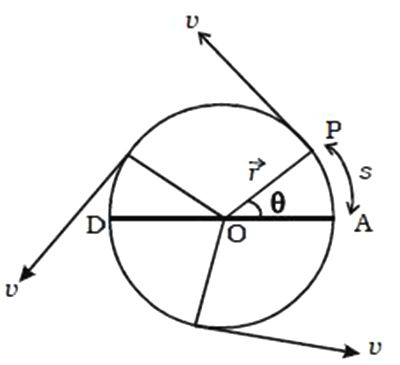# Explain Uniform Circular Motion

The revolution of the Earth around the Sun, rotating flywheel, electrons revolving around the nucleus, spinning top, the motion of a fan blade, revolution of the moon around the Earth etc. are some examples of circular motion. In all cases, the bodies or particles travel in a circular path. So, it is necessary to understand the motion of such bodies.

When a particle moves on a circular path with a constant speed, then its motion is known as uniform circular motion in a plane. The magnitude of velocity in circular motion remains constant but the direction changes continuously. Let us consider a particle of mass m moving with a velocity v along the circle of radius r with center O as shown in Figure.Fig: Uniform Circular Motion

P is the position of the particle at a given instant of time such that the radial line OP makes an angle 6 with the reference line DA. The magnitude of the velocity remains constant, but its direction changes continuously. The linear velocity always acts tangentially to the position of the particle (i.e) in each position; the linear velocity v is perpendicular to the radius vector r.